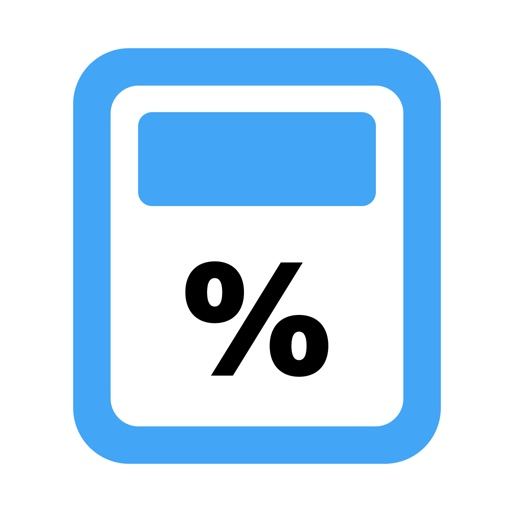# The Percentage Calculator

by Nikolay Iliev

## Screenshots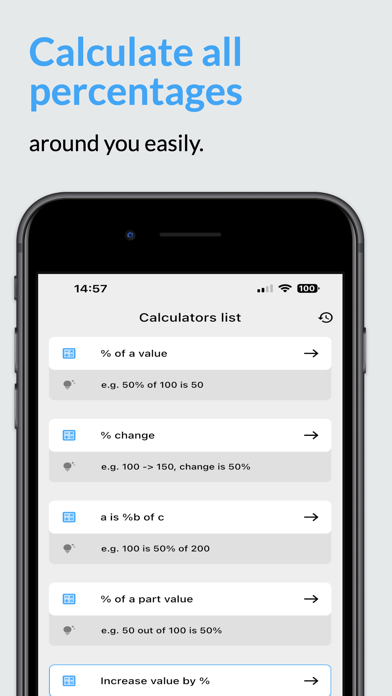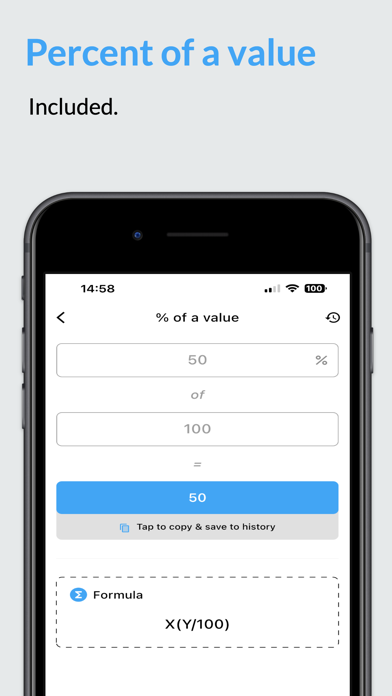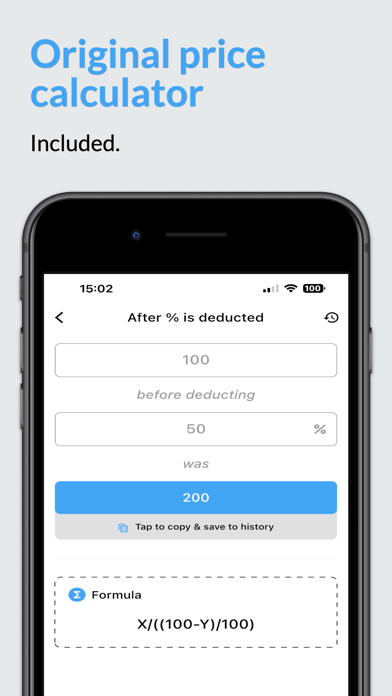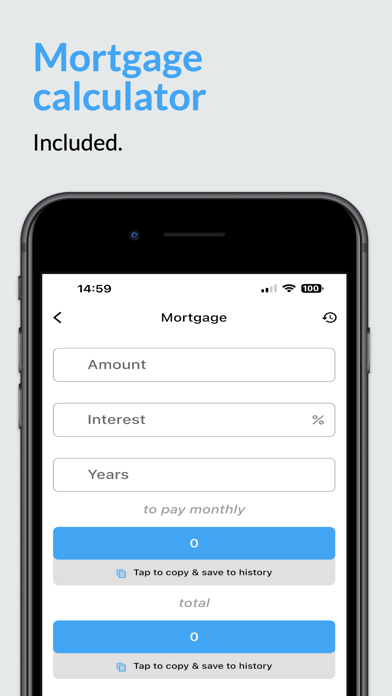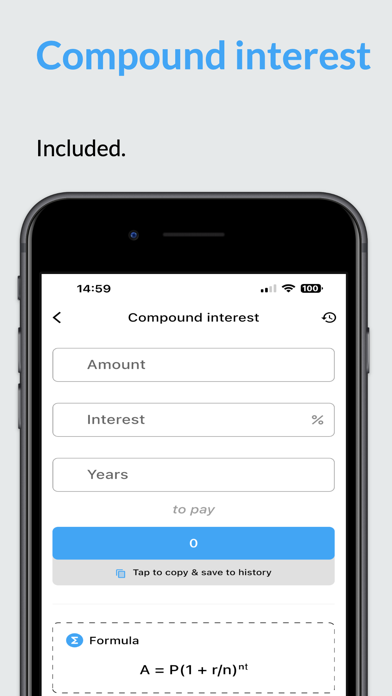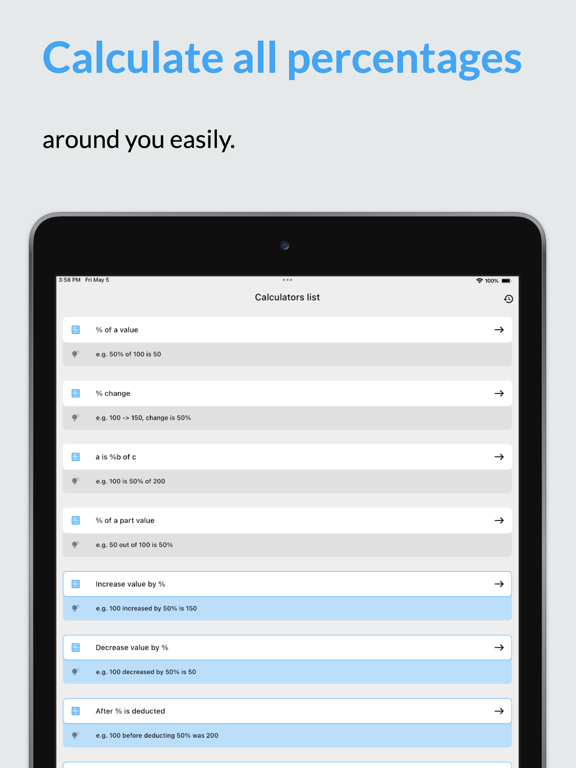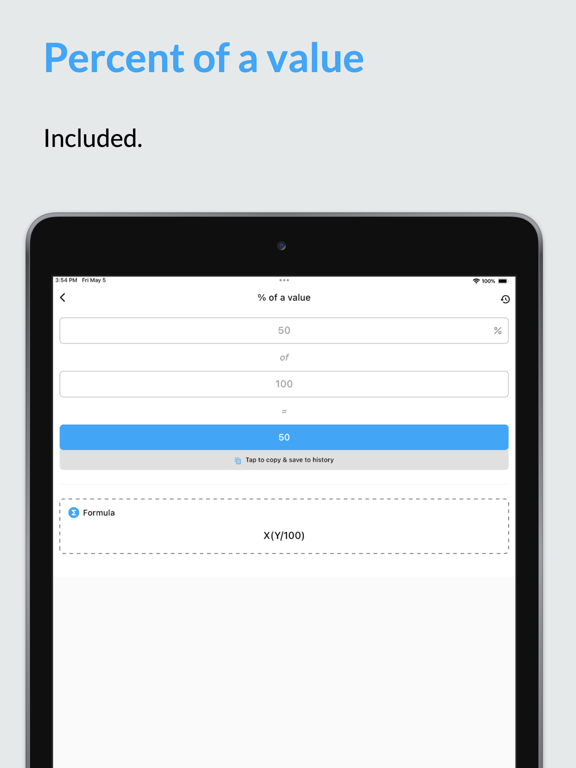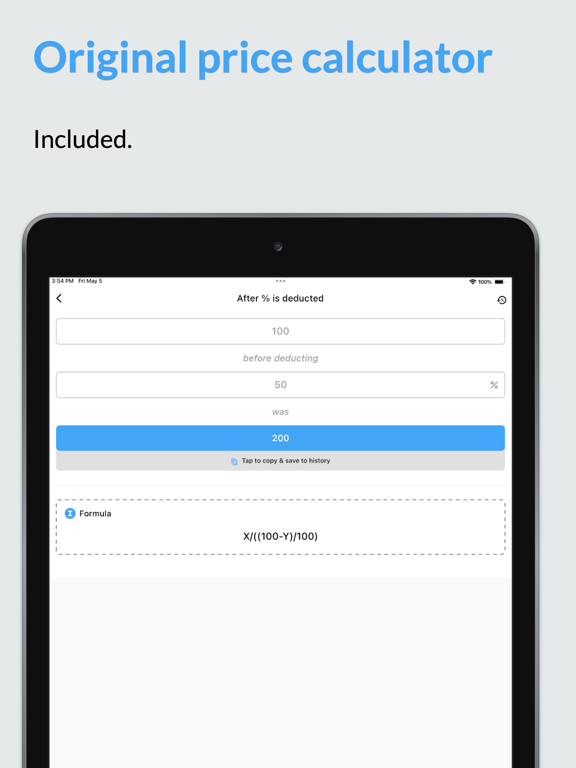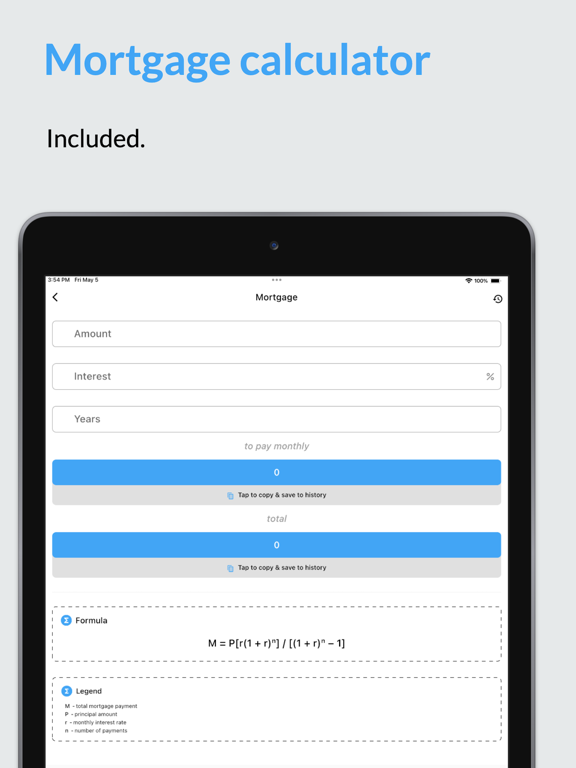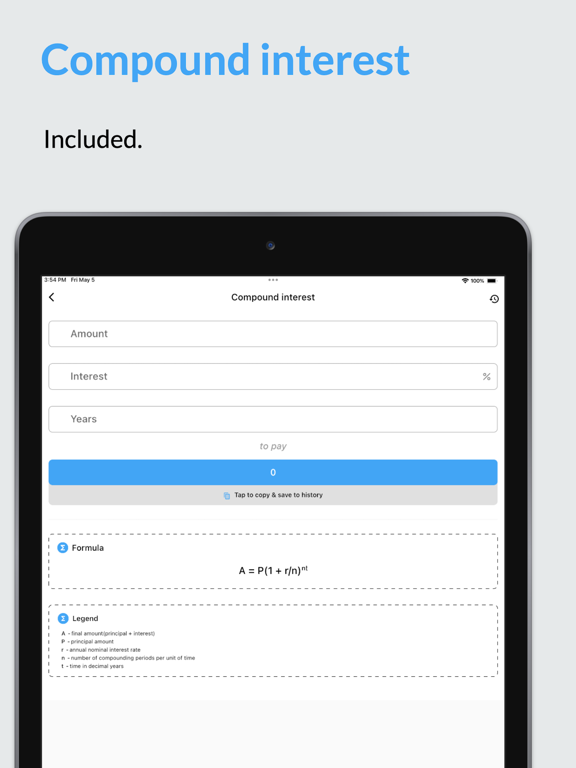The Percentage Calculator is an all-in-one iOS app designed to make percentage calculations easier and quicker. Whether you're a student, teacher, businessman, or anyone who deals with numbers, this app will save you time and energy by providing quick solutions to your percentage problems.

► WHAT TO EXPECT FROM THIS QUICK PERCENTAGE CALCULATOR?
1. Percentage of a concrete number: This calculator helps you find the percentage of a number. Simply enter the number and the percentage, and the app will instantly calculate the result.
2. Change in the percentage: This free discount calculator helps you find the percentage increase or decrease in a number. Whether it's a discount or a price hike, this calculator will tell you exactly how much the percentage has changed.
3. Percentage increase or decrease: This all-in-one calculator to calculate discounts helps you find the percentage increase or decrease between two numbers.
4. Reverse percentages: This free calculator helps you find the original number after a percentage increase or decrease. Simply enter the percentage and the new number, and the app will calculate the original number.
5. Interest rate calculation: This free percentage calculator helps you calculate the interest rate on a loan or investment. Whether you're borrowing or investing, this calculator will help you determine the interest rate.
6. Discount percentage calculation: This quick discount calculator helps you calculate the discount percentage on a product or service.
7. Percentage in decimal form: You can easily convert percentages to decimal form. Whether you're calculating tax or interest, this calculator will help you convert percentages to decimal form.
8. Percentage of a part value: This free interest rate calculator helps you find the percentage of a part value in relation to the whole. Whether you're calculating the percentage of a total cost or a portion of a product, this calculator will help you determine the percentage.
9. Fraction in percentage form: Using this free percentage calculator you can convert fractions to percentages. Whether you're calculating measurements or solving math problems, this calculator will help you convert fractions to percentages.
10. Percentage point between percentages: This calculator helps you find the percentage point difference between two percentages. Whether you're measuring growth or comparing statistics, this calculator will help you determine the percentage point difference.
11. Compound interest: This loan calculator helps you calculate the compound interest on a loan or investment. Whether you're making monthly payments or investing in a long-term project, this calculator will help you determine the compound interest.
12. Mortgage: This free debt and mortgage calculator helps you calculate the monthly mortgage payments on a property. Whether you're looking to buy a house or invest in a property, this calculator will help you determine the monthly mortgage payments.

►HOW DOES THIS PERCENTAGE CALCULATOR STAND OUT IN THE COMPETITION?
The Percentage Calculator app provides many benefits to users, including
1. Time-saving: This free interest rate and discount calculator app provides quick and accurate results for all your percentage calculations.
2. Versatility: The all-in-one percentage calculator offers a range of calculators to meet all your percentage calculation needs, making it a versatile tool for students, teachers, businessmen, and anyone who deals with numbers.
3. It’s Free to use: Users can benefit from the first 4 calculators free of charge (with ads), and we offer monthly subscriptions and lifetime subscriptions for complete access to all the available calculators.